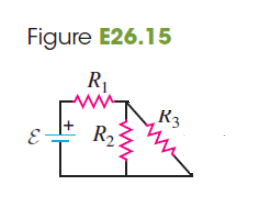# Problem: In the circuit of Fig. E26.15, each resistor represents a light bulb. Let R1 = R2 = R3 = 4.50 Ω and ε = 9.00 V. (a) What is the current in each of the remaining bulbs R1, R2, and R3?(b) What is the power dissipated in each of the remaining bulbs?

###### FREE Expert Solution

Equivalent resistance for 2 resistors in parallel:

$\overline{){{\mathbf{R}}}_{{\mathbf{eq}}}{\mathbf{=}}\frac{{\mathbf{R}}_{\mathbf{1}}{\mathbf{R}}_{\mathbf{2}}}{{\mathbf{R}}_{\mathbf{1}}\mathbf{+}{\mathbf{R}}_{\mathbf{2}}}}$

Equivalent resistance for resistors in series:

$\overline{){{\mathbf{R}}}_{{\mathbf{eq}}}{\mathbf{=}}{{\mathbf{R}}}_{{\mathbf{1}}}{\mathbf{+}}{{\mathbf{R}}}_{{\mathbf{2}}}{\mathbf{+}}{\mathbf{.}}{\mathbf{.}}{\mathbf{.}}{\mathbf{+}}{{\mathbf{R}}}_{{\mathbf{n}}}}$

Current:

$\overline{){\mathbf{i}}{\mathbf{=}}\frac{\mathbf{V}}{\mathbf{R}}}$

Power:

$\overline{){\mathbf{P}}{\mathbf{=}}{{\mathbf{i}}}^{{\mathbf{2}}}{\mathbf{R}}}$

(a)

Req = R + (R)(R)/(R + R) = R + R/2 = 1.5R

Req = (1.5)(4.50) = 6.75Ω

85% (249 ratings)###### Problem Details

In the circuit of Fig. E26.15, each resistor represents a light bulb. Let R1 = R2 = R3 = 4.50 Ω and ε = 9.00 V.(a) What is the current in each of the remaining bulbs R1, R2, and R3?

(b) What is the power dissipated in each of the remaining bulbs?